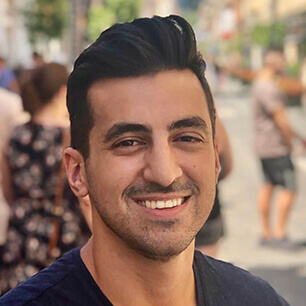# Conquer OAT Quantitative Reasoning with OAT Bootcamp

Get 10 practice tests, 1,152 questions, and 950+ videos.

### What's the OAT Quantitative Reasoning section like?

40 math questions are presented and must be answered in 45 minutes.

The math on the OAT is the same math you practiced in high school. It focuses on algebra, word problems, and data analysis. There is no calculus. The best way to prepare for this section is to just jump in and practice using the QR question bank. Do about 20 questions a day, following the same techniques you’ve been using to answer practice questions so far. If you haven’t practiced math in a while, check out our QR Academy, it will help cover the foundations. Finally, do the practice tests at the end.

QR is the only section you’ll have an on-screen calculator. Math questions on OAT Bootcamp have the exact same calculator, click the Exhibit button in a math question to bring it up.

Since this is a time-intensive test, we will tackle this section in two runs. First, go through the QR section and answer all of the easy questions. If you see a problem and you immediately know how to do it, solve it right there. If a problem looks difficult or you’re not sure of how to solve it, guess, mark, and move on. Second, after you answer all the easy questions, move on to the marked questions. This way, you’ll get all the easy points and at least put down an answer to all the questions.